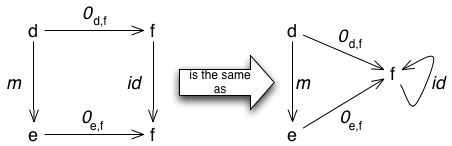# Zeros in Category Theory

Things are a bit busy at work on my real job lately, and I don’t have time to put together as detailed a post for today as I’d like. Frankly, looking at it, my cat theory post yesterday was half-baked at best; I should have held off until I could polish it a bit and make it more comprehensible.
So I’m going to avoid that error today. Since we’ve had an interesting discussion focusing on the number zero, I thought it would be fun to show what zero means in category theory.
There are two zeros it category theory: the zero object, and the zero arrow.
Zero Objects
————–
The zero object is easier. Suppose we’ve got a category, C. Suppose that C has a *terminal object* – that is, an object t for which other object x in C has *exactly* *one* arrow f : x → t. And suppose that C also has an *initial object*: that is, an object i for which every object x in C has *exactly one* arrow, f : i → x. If C has both an initial object i, and a terminal object t, *and* i = t, then it’s a *zero object* for the category. A category can actually have *many* zero objects. For example, in the category of groups, any *trivial* group (that is, a group which contains only one element) is a zero object in the category of groups. For an intuition of why this is called “zero” think of the number zero. It’s a strange little number that sits dead in the middle of everything. It’s the central point on the complex plane, it’s the center of the number line. A zero *object* sits in the middle of a category: everything has exactly one arrow *to* it, and one arrow *from* it.
Zero Arrows
————-
The idea of a zero *arrow* comes roughly from the basic concept of a zero *function*. A zero function is a function f where for all x, f(x) = 0. One of the properties of a zero function is that composing a zero function with any other function results in a zero function. (That is, if f is a zero function, f(g(x))) is also a zero function.)
A zero morphism in a category C is part of a set of arrows, called the *zero family* of morphisms of C, where composing *any* morphism with a member of the zero family results in a morphism in the zero family.
To be precise: suppose we have a category C, and for any pair b of objects a and b ∈ Obj(C), there is a morphism 0a,b : a → .
The morphism 0d,e must satisfy one important property:
For any two morphisms m : d → e, and n : f → g, the following diagram must commute:To see why this means that any composition with a 0 arrow will give you a zero arrow, look at what happens when we start with an ID arrow. Let’s make n : f → g an id arrow, by making f=g. We get the following diagram:So – any zero arrow composed with an id arrow is a zero arrow. Now use induction to step up from ID arrows by looking at one arrow composed with an ID arrow and a zero arrow. You’ve still got a zero. Keep going – you’ll keep getting zeros.
Zero arrows are actually very important buggers: the algebraic and topographical notions of a kernel can be defined in category theory using the zero morphisms.
One last little note: in general, it looks like the zero objects and the zero morphisms are unrelated. They’re not. In fact, if you have a category with a zero object *and* a family of zero morphisms, then you can find the zero morphisms by using the zero object. For any objects a and b, 0a,b = (a → 0) º (0 → b).

## 0 thoughts on “Zeros in Category Theory”

1.Blake Stacey

The zero arrow, invented by Mayan category-theoreticians around the year 700. . . .

2.Chad Groft

One last little note: in general, it looks like the zero objects and the zero morphisms are unrelated. They’re not. In fact, if you have a category with a zero object and a family of zero morphisms, then you can find the zero morphisms by using the zero object. For any objects a and b, 0_{a,b} = (a → 0) º (0 → b).

More that that; it looks like, given a zero object 0, the family of arrows 0_{a,b} = (a → 0) º (0 → b) are automatically zero morphisms. Plugging these into the commutative square above, and using the fact that 0 is both initial and terminal, both directions along the square should be 0_{d,g}. Is that correct?

3.Zero

Excellent. I’d love to see more posts about zero. It reminds me of my love for Xeno’s Dichotomy. I’m off to check out the Zero Saga.
Posted by: Gabe | July 21, 2006 03:46 PM
****************************************
Size 101 So how big am I?
IMO, there are two infinities, big & small.
Infinity “big” is plus. Infinity “small” is minus.
Isa 40:12 ¶ Who hath measured the waters in the hollow
of his hand, and meted out heaven with the span, and comprehended
the dust of the earth in a measure, and weighed the mountains in scales, and the hills in a balance?
me………………a speck on earth
earth……………a speck in the milky way
milky way…….a speck in the universe
universe……….a speck in God’s pocket
From my perspective:
Anything smaller than me is small.
Anything bigger than me is big.
Zero